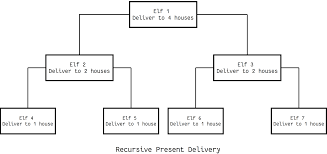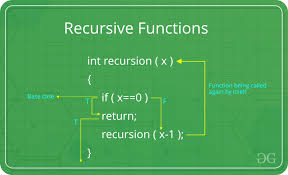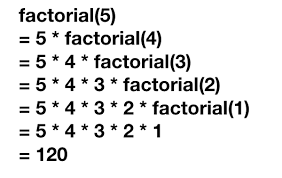Python Recursion

In this tutorial we are going to learn about recursion concept. To use this, we have to use some recursion function(that functions which Call Itself).

What is recursion in Python?Recursion is a way of programming or coding a problem, in which a function calls itself one or more times in its body. Usually, it is returning the return value of this function call. If a function definition full-fills the condition of recursion, we call this function a recursive function.

------------------------------------OR-------------------------------------------

Recursion is the process of defining something in terms of itself.

The adjective "recursive" originates from the Latin verb "recurrere", which means "to run back". And this is what a recursive definition or a recursive function does: It is "running back" or returning to itself. Most people who have done some mathematics, computer science or read a book about programming will have encountered the factorial, which is defined in mathematical terms as

n! = n * (n-1)!, if n > 1 and f(1) = 1

For Example:-
A physical world example is assume take two parallel mirrors facing each other. Any object in between them would be reflected recursively.

Python Recursive FunctionIn Python, we know that a function can call other functions. It is even possible for the function to call itself. These type of construct are termed as recursive functions.

Following is an example of a recursive function to find the factorial of an integer:-
• Factorial of a number is the product of all the integers from 1 to that number.
• For example, the factorial of 8 (denoted as 8!) is 1*2*3*4*5*6*7*8 = 40, 320.

Example of a recursive function in Python Programdef fac(x):

if x == 1:
return 1
else:
return (x * fac(x-1))

num = 8
print("The factorial of", num, "is", fac(num))

In the above example, fac() is a recursive function as it calls itself.

When we call this function with a positive integer, it will recursively call itself by decreasing the number.

Each function calls multiples the number with the factorial of the number below it until it is equal to one.Our recursion ends when the number reduces to 1. This is called the base condition.

Every recursive function must have a base condition that stops the recursion or else the function calls itself infinitely.

The Python interpreter limits the depths of recursion to help avoid infinite recursions, resulting in stack overflows.

By default, the maximum depth of recursion is 1000.

·         Make the code look clean and elegant for the Recursive Functions..
·         Make complex task could be broken down into simpler sub-problems using recursion.
·         Sequence generation is easier with recursion than using some nested iteration.